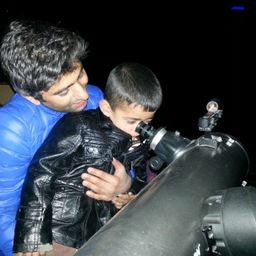Gravitation

### Discussion

You must be signed in to discuss.
##### Top Physics 101 Mechanics Educators##### Andy C.

University of Michigan - Ann Arbor##### Marshall S.

University of WashingtonLectures

Join Bootcamp

### Video Transcript

we know that Al equals l equals proper length. Times square room, toe one minus. We screen everybody by C square. Okay, on DDE. In this case, L is equal to 28 minus 0.15 and 28 minus 2.15 is equal to 27 point 85 Get five. Now let's substitute values well, that we have and we do. So 27 0.85 is equal to Ah, proper length is 28. Multiply by squared Rudolph one minus B squared, divided by C. Suite one minus. We squared, divided by C square. Now let's stick square on both sides. 27.85 What screen is equal to 28 square. Multiply by one minus. We squared, divided by C square and we have ah to one minus B squared. Divided by C square is equal to, um 27 point 85 All this were divided by 28 square on DDE and further minus, uh, we squared, divided by C square circle too. Lost 700 75 point six 225 divided by See when they to four minus one. And from this we have re divided by sea, is equal to 0.1 cereal tree and V equals zero point 103 times the speed of light.#### Topics

Gravitation

##### Top Physics 101 Mechanics Educators##### Andy C.

University of Michigan - Ann Arbor##### Marshall S.

University of WashingtonLectures

Join Bootcamp